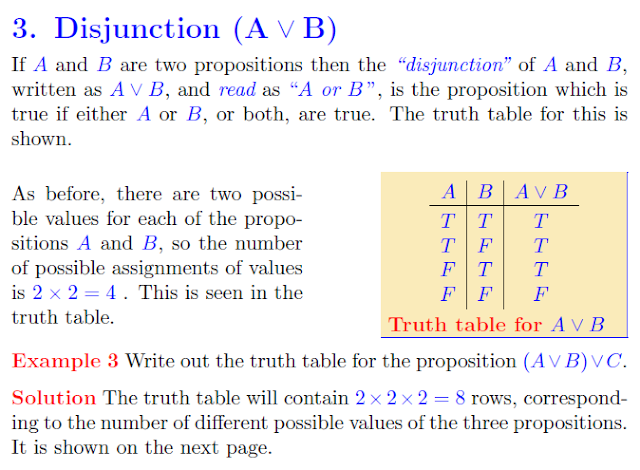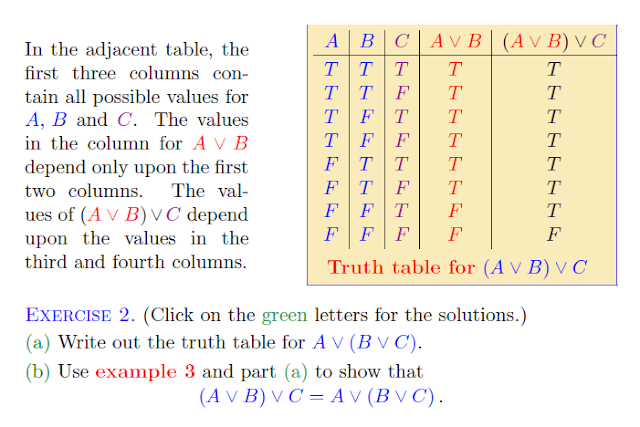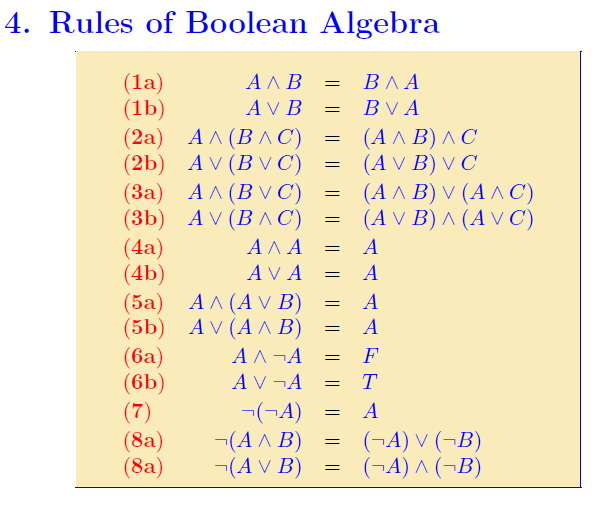# SCC Education

## Boolean algebra ,negation,truth table,logical equivalent,conjunction,disjunctive,rule of Boolean algebra,introduction-of-hyperbolic-function
solving-models-related-to-ordinary differential equation
laplace-transform unit-step
the-inverse-hyperbolic-sine-inverse
multiple-integrals
l hopitals-rule
rules-of-differentiation partial
numerical-integration
critical-points-and-point-of-inflection
asymptotes
differentiation-of-vector
summary-of-convergence-test
runga-kutta-method
laplace-formulas
volume-of-solid-of-revolution
complex-integration
vector-calculus-questions
laplace-transforms-question
differential-equation-of-first-order
the-trapezium-rule
limit-continuity differentiability
numerical-differentiation